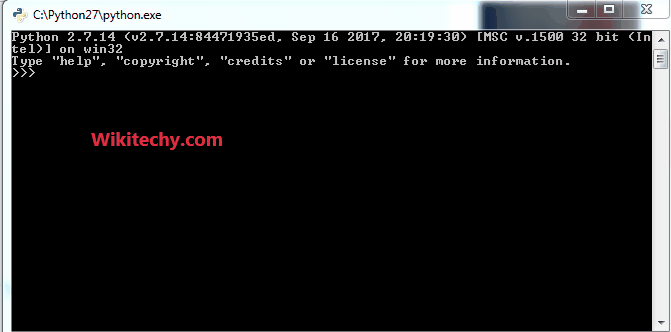# python tutorial - Python Function | Operator Functions in Python | Part 1 - learn python - python programming

Python has predefined functions for many mathematical, logical, relational, bitwise etc operations under the module “operator”.

1. add(a, b) :- This functions returns addition of the given arguments.
Operation – a + b.

2. sub(a, b) :- This functions returns difference of the given arguments.
Operation – a – b.

3. mul(a, b) :- This functions returns product of the given arguments.
Operation – a * b.python - Sample - python code :Learn Python - Python tutorial - python operatorfun - Python examples - Python programs

python tutorial - Output :

```The addition of numbers is :7
The difference of numbers is :1
The product of numbers is :12
```

4. truediv(a,b) :- This functions returns division of the given arguments.
Operation – a / b.

5. floordiv(a,b) :- This functions also returns division of the given arguments. But the value is floored value i.e. returns greatest small integer.
Operation – a // b.

6. pow(a,b) :- This functions returns exponentiation of the given arguments.
Operation – a ** b.

7. mod(a,b) :- This functions returns modulus of the given arguments.
Operation – a % b.

python - Sample - python code :

python tutorial - Output :

```The true division of numbers is : 2.5
The floor division of numbers is : 2
The exponentiation of numbers is : 25
The modulus of numbers is : 1
```

8. lt(a, b) :- This function is used to check if a is less than b or not. Returns true if a is less than b, else returns false.
Operation – a < b.

9. le(a, b) :- This function is used to check if a is less than or equal to b or not. Returns true if a is less than or equal to b, else returns false.
Operation – a <= b.

10. eq(a, b) :- This function is used to check if a is equal to b or not. Returns true if a is equal to b, else returns false.
Operation – a == b.

python - Sample - python code :

python tutorial - Output :

```3 is not less than 3
3 is less than or equal to 3
3 is equal to 3
```

11. gt(a,b) :- This function is used to check if a is greater than b or not. Returns true if a is greater than b, else returns false.
Operation – a > b.

12. ge(a,b) :- This function is used to check if a is greater than or equal to b or not. Returns true if a is greater than or equal to b, else returns false.
Operation – a >= b.

13. ne(a,b) :- This function is used to check if a is not equal to b or is equal. Returns true if a is not equal to b, else returns false.
Operation – a != b.

python - Sample - python code :

python tutorial - Output :

```4 is greater than 3
4 is greater than or equal to 3
4 is not equal to 3
```

Wikitechy tutorial site provides you all the learn python , python programming online training , python course online , python programming course